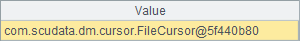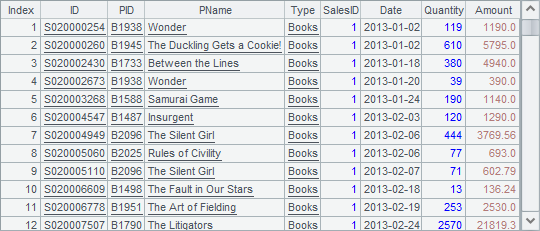• 集算器
教程
函数参考
用户参考
外部库使用指南
数据文件工具用户参考
DQL教程
集群管理器使用说明
SPL命令执行器用户参考
• 润乾报表
教程
填报教程
分析教程
报表中心教程
用户参考
程序员参考
高级指南
通用查询
DQL部署集成手册
• 易明建模
用户参考
json参数说明
• 润乾官网

# 游标聚合运算

## 游标的分组聚合运算

 A 1 =file("Order_Wines.txt") 2 =file("Order_Electronics.txt") 3 =file("Order_Foods.txt") 4 =file("Order_Books.txt") 5 =[A1:A4].(~.cursor@t()) 6 =A5.mergex(Date) 7 =A6.groups(Type;count(~):OrderCount,max(Amount):MaxAmount, sum(round(decimal(Amount),2)):Total)

A7中，分种类统计了各类商品的订单总数、最大交易金额及总金额。需要注意的是，在大数据计算中，双精度数据的精度往往不够，此时需要用decimal() 将数据转换为数值类型计算。在游标的分组聚合运算中，所有数据都会被遍历一次，遍历时，对每行记录执行它在相应分组的聚合计算，在一次遍历中可以同时计算多个聚合值，在遍历完成后游标会自动关闭。

A7中结果如下：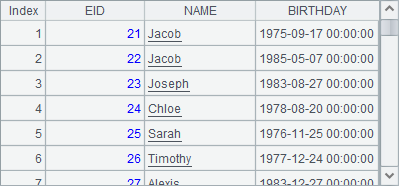A 1 =file("Order_Wines.txt") 2 =file("Order_Electronics.txt") 3 =file("Order_Foods.txt") 4 =file("Order_Books.txt") 5 =[A1:A4].(~.cursor@t()) 6 =A5.mergex(Date) 7 =A6.groups(Type;top(3,Amount):MinAmount,top(3,-Amount):MaxAmount)

A7中计算了每类商品订单中，总价最低的3笔订单金额和总价最高的3笔订单金额，注意其中计算最高3笔金额时，在聚合函数中添加负号，用top(3,-Amount)。结果如下：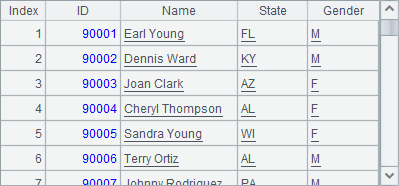A 1 =file("Order_Wines.txt") 2 =file("Order_Electronics.txt") 3 =file("Order_Foods.txt") 4 =file("Order_Books.txt") 5 =[A1:A4].(~.cursor@t()) 6 =A5.mergex(Date) 7 =A6.groups(;count(~):Count,sum(round(decimal(Amount),2)):TotalAmount)

A7中结果如下：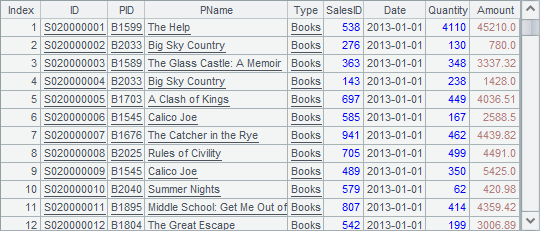## 多个游标的聚合运算

 A 1 =file("Order_Wines.txt") 2 =file("Order_Electronics.txt") 3 =file("Order_Foods.txt") 4 =file("Order_Books.txt") 5 =[A1:A4].(~.cursor@t()) 6 =A5.(~.groups(Date;count(~):Count,sum(round(decimal(Amount),2)):TotalAmount))

A6中，对各个文件游标分别分组聚合，计算出每类商品每天的聚合统计结果，如下：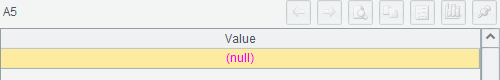A 1 =file("Order_Wines.txt") 2 =file("Order_Electronics.txt") 3 =file("Order_Foods.txt") 4 =file("Order_Books.txt") 5 =[A1:A4].(~.cursor@t()) 6 =A5.(~.groups(Date;count(~):Count,sum(round(decimal(Amount),2)):TotalAmount)) 7 =A6.merge(Date) 8 =A7.groups(Date;sum(Count):Count,sum(round(decimal(TotalAmount),2)):TotalAmount)

A8中的汇总结果如下：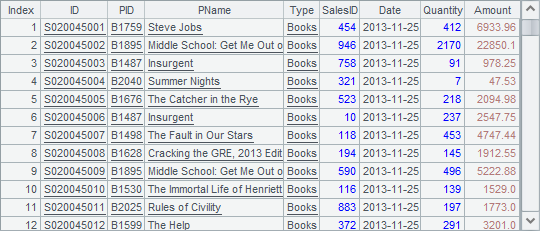A 1 =file("Order_Wines.txt") 2 =file("Order_Electronics.txt") 3 =file("Order_Foods.txt") 4 =file("Order_Books.txt") 5 =[A1:A4].(~.cursor@t()) 6 =A5.mergex(Date) 7 =A6.groups(Date;count(~):Count,sum(round(decimal(Amount),2)):TotalAmount)

## 大数据结果集

 A 1 =file("Order_Wines.txt") 2 =file("Order_Electronics.txt") 3 =file("Order_Foods.txt") 4 =file("Order_Books.txt") 5 =[A1:A4].(~.cursor@t()) 6 =A5.conjx() 7 =A6.groupx(Date;count(~):Count,sum(round(decimal(Amount),2)):TotalAmount;100) 8 =A7.fetch(100) 9 =A7.fetch(100) 10 =A7.fetch(100) 11 =A7.fetch(100)

A6中，各个游标中数据依次连接，并非按日期排序。在A7中分组聚合后，将返回游标，而非序表，这样在A8~A11中即可分步读取数据，数据如下：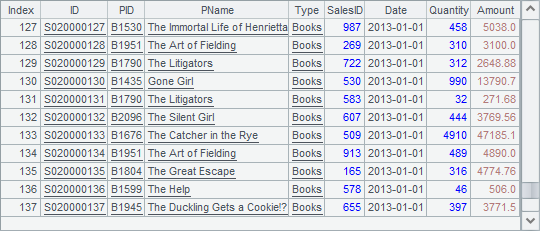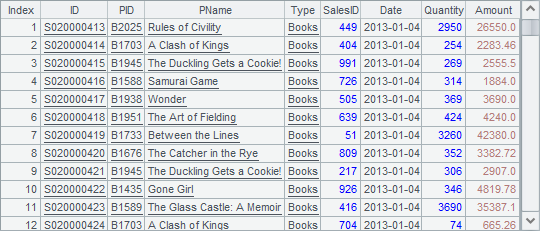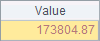A7中，使用groupx函数，将数据按日期分组聚合，并设定缓冲行数为100A7中的groupx函数中，最后一个参数100，表示内存中最多容纳100条数据，每100条数据就需缓存数据。这个参数在实际应用中是不需要设定的，集算器在执行缓存时会自动估算当前内存可容纳的数据条数，在必须的情况下才缓存数据。这样，在A7执行时，将边读取游标A6中的数据，边拆分聚合，每出现100行聚合结果，即缓存到一个临时文件中，以此类推。而A7中的计算结果，是由这些临时文件构成的文件组游标：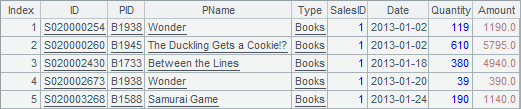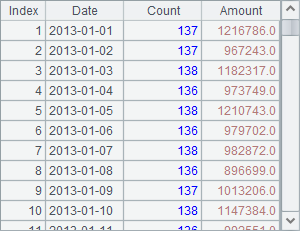A 1 =file("temp/tmpdata1372633253707222252").import@b() 2 =file("temp/tmpdata2669923936558108709").import@b() 3 =file("temp/tmpdata3937021394605697131").import@b() 4 =file("temp/tmpdata8767777617311441973").import@b()

A1A3中读出的数据分别如下：